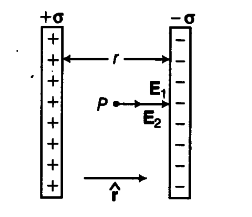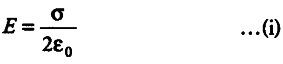# Use Gauss’ law to derive the expression

Use Gauss’ law to derive the expression for the electric field between two uniformly charge parallel sheets with surface charge densities a and - a respectively.

Let us consider two uniformly charge parallel sheets carrying surface charge densities +0> and — CT respectively and are separated by a small distance from each other.By Gauss’ law, it can be proved that, electric field intensity due to a uniformly charged infinite plane sheet as nearby is given byThe electric field is directed normally outward from the plane sheet, if nature of charge on sheet is positive and normally inward, if charge is of negative nature. Let ? represents unit vector directed from positive plate to negative plate.
Now, electric field intensity (EFI) at any point P between the two plates is given by